## 3. 3. 1 Standard Formulation

In contrast to finite elements or finite volumes, shape functions do not have local support but they are non-zero throughout the simulation domain. Normally, basis functions are defined in a manner that they are defined by functions having boundary facets as local support. These facets are incident with a common vertex. In the two-dimensional case, the facets are (boundary) edges incident with a boundary vertex.

The typical formulation of a boundary element scheme can be written in the following manner (Galerkin formulation)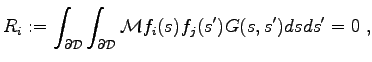(3.46)

where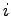and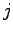are indices of vertices. For each vertex an equation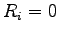is assembled. The fundamental solution  function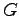denotes a fundamental solution of the respective differential operator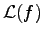: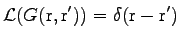(3.47)

The parameters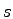and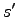denote the position at the boundary surface or curve. The linear boundary operator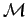can be written as linear combination in the following manner.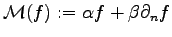(3.48)

Michael 2008-01-16